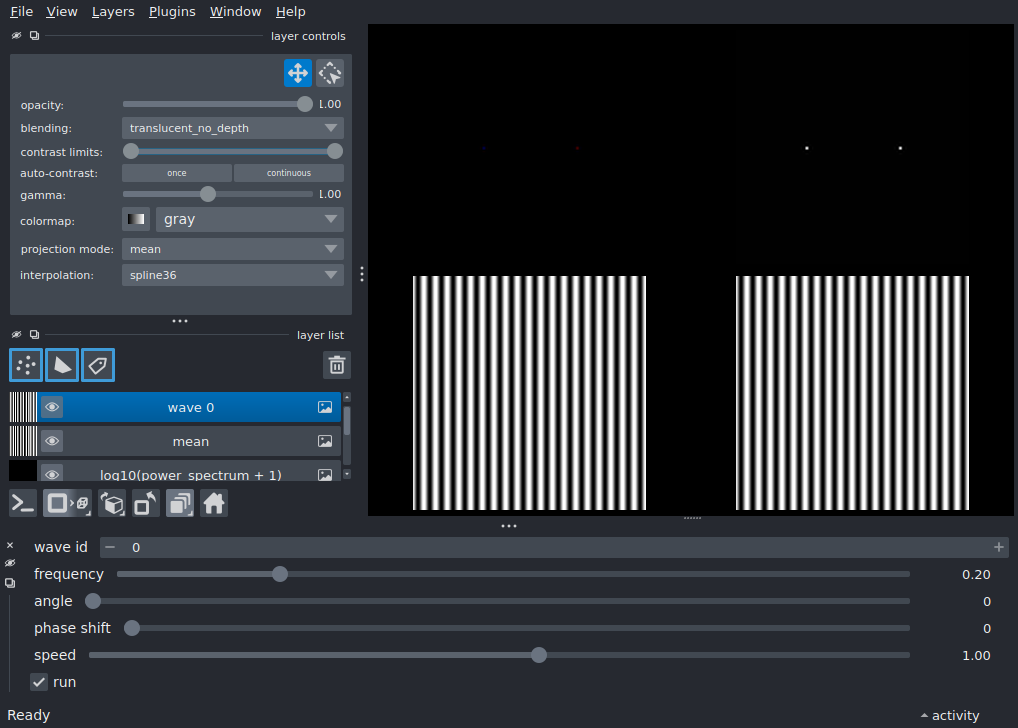# Fourier transform playground¶

Generate an image by adding arbitrary 2D sine waves and observe how the fourier transform’s real and imaginary components are affected by the changes. Threading is used to smoothly animate the waves.

Tags: interactivity, gui```from time import sleep, time

import numpy as np
from magicgui import magic_factory
from scipy.fft import fft2, fftshift

import napari

IMAGE_SIZE = 100
FPS = 20

# meshgrid used to calculate the 2D sine waves
x = np.arange(IMAGE_SIZE) - IMAGE_SIZE / 2
X, Y = np.meshgrid(x, x)

def wave_2d(frequency, angle, phase_shift):
"""Generate a 2D sine wave based on angle, frequency and phase shift."""
wave = 2 * np.pi * (X * np.cos(angle) + Y * np.sin(angle)) * frequency
return np.sin(wave + phase_shift)

# set up viewer with grid-mode enabled
viewer = napari.Viewer()
viewer.grid.enabled = True

def update_layer(name, data, **kwargs):
"""Update a layer in the viewer with new data.

If data is None, then the layer is removed.
If the layer is not present, it's added to the viewer.
"""
if data is None:
if name in viewer.layers:
viewer.layers.pop(name)
viewer.reset_view()
elif name not in viewer.layers:
viewer.reset_view()
else:
viewer.layers[name].data = data

def combine_and_set_data(wave_args):
"""Merge 2D waves, calculate the FT and update the viewer.

The wave phases are offset by the current time multiplied by an
arbitrary speed value; this generates an animated wave if called repeatedly.
"""
if not wave_args:
# this happens on yielding from the thread, no need to update anything
return

t = time()
waves = {
wave_id: wave_2d(frequency, angle, phase_shift + t * speed * 100) if frequency else None
for wave_id, (frequency, angle, phase_shift, speed) in wave_args.items()
}

to_add = [d for d in waves.values() if d is not None]
ft = fftshift(fft2(mean))
power_spectrum = abs(ft)
phase = np.angle(ft) * power_spectrum
power_spectrum = np.log10(power_spectrum + 10)
else:
mean = power_spectrum = phase = None

# for visualisation, it's clearer to use:
# phase * ps instead of phase
# and log10(ps + 1) instead of ps
update_layer('phase * power_spectrum', phase, colormap=('blue', 'black', 'red'))
update_layer('log10(power_spectrum + 1)', power_spectrum)
update_layer('mean', mean)

for name, data in waves.items():
update_layer(f'wave {name}', data)

def update_viewer():
# keep track of each wave in a dictionary by id, this way we can modify/remove
# existing waves or add new ones
wave_args = {}
new_params = None
while True:
sleep(1 / FPS)
# this receives new_params from thread.send() and yields {} for the `yielded` callback
new_params = yield wave_args
if new_params is not None:
# note that these come from thread.send() in moving_wave()!
wave_id, *args = new_params
wave_args[wave_id] = args
yield wave_args

# start the thread responsible for updating the viewer

@magic_factory(
auto_call=True,
frequency={'widget_type': 'FloatSlider', 'min': 0, 'max': 1, 'step': 0.01},
angle={'widget_type': 'Slider', 'min': 0, 'max': 180},
phase_shift={'widget_type': 'Slider', 'min': 0, 'max': 180},
speed={'widget_type': 'FloatSlider', 'min': -10, 'max': 10, 'step': 0.1},
)
def moving_wave(
wave_id: int = 0,
frequency: float = 0.2,
angle: int = 0,
phase_shift: int = 0,
speed: float = 1,
run=True,
):
"""Send new parameters to the listening thread to update the 2D waves.

The `run` checkbox can be disabled to stop sending values to the thread
while changing parameters.
"""
if run:

wdg = moving_wave()

# add the widget to the window and run it once